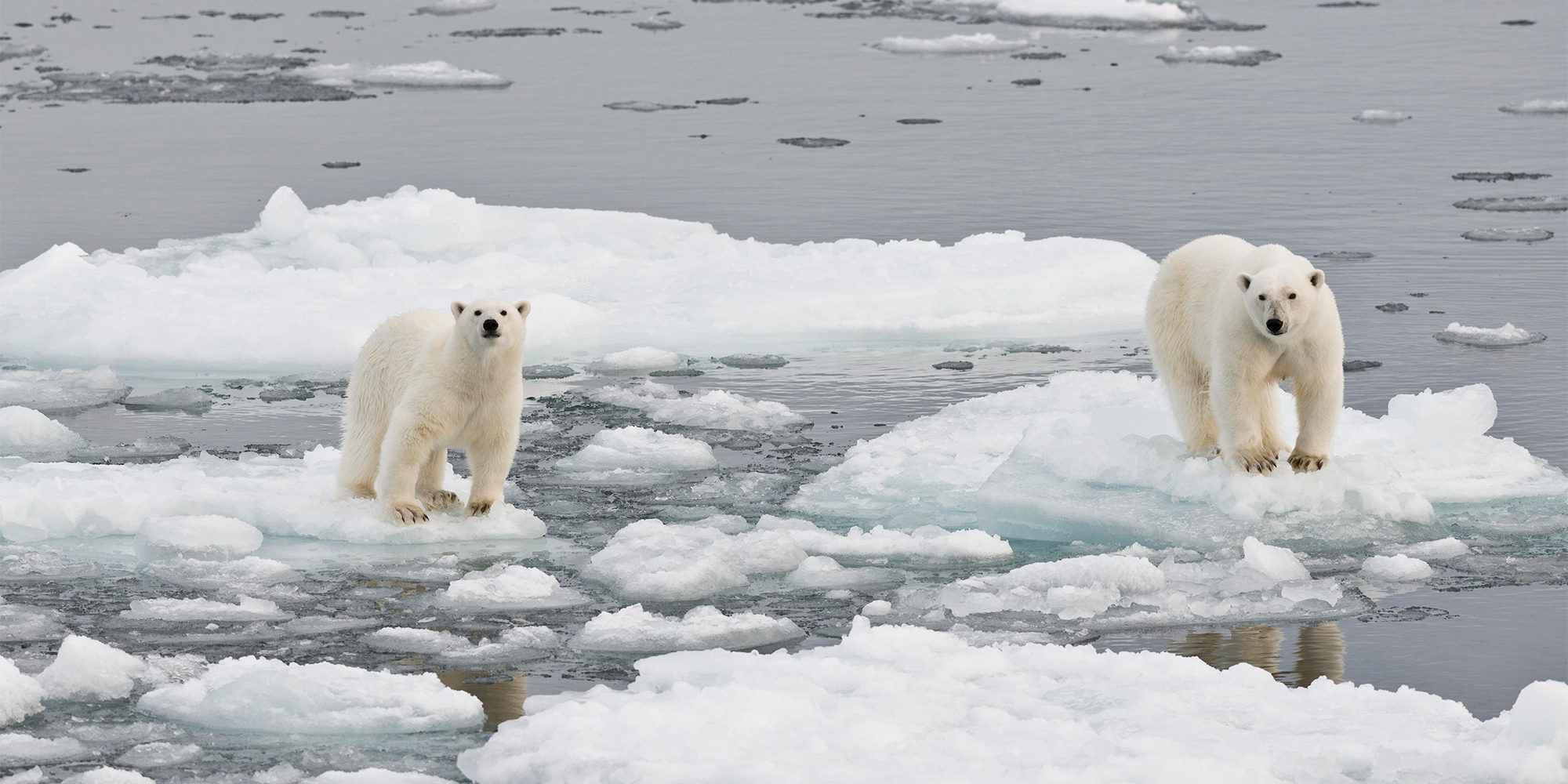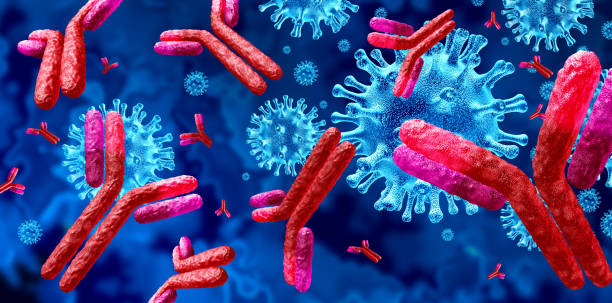### Animal Physiology for IUP (BISB211403)Animal physiology is biological sub-discipline that tries to understand how animals function in physical and chemical terms. Physiologists study the principles, processes, and mechanisms that underpin biological activity, including cellular systems of molecular interactions and organismal systems of interacting cells and tissues. They also look at how these functions are influenced by the organism's evolutionary history, environment, size, shape, and structure, as well as physical and chemical laws. The common goal of physiologists is to comprehend the integrated functioning of the entire body, as well as the methods by which physiological activities are regulated.

This introductory course will attempt to reflect this scope and convey the enthusiasm of physiological research by using a variety of conceptual and experimental approaches. In a comparative approach, this course will use examples from all around the animal kingdom. The purpose of this course is to give students a firm foundation in the cellular basis of animal physiology, with a focus on homeostasis and the endocrine system. The relevance of homeostasis and the endocrine system in the reproductive, osmoregulatory, and digestive systems of animals will be discussed further in this course. Furthermore, we will make an effort to demonstrate the importance of experimentation in the development of our knowledge about living animals.

### Animal Systematics IUP (BIB 21101)

Animal Systematics is a compulsory course on animal diversity, especially in Indonesia. This course covers principles in systematics, theory and practices in animal taxonomy, integrating phylogeny and taxonomy to represent relationships among animals. This course is fundamental for other biological sciences such as genetics, ecology, conservation and animal culture.

### English For Biology IUP (BISB211101)

English for Biology (BISB211101)

IUP Class

### Evolution IUP (BIB 40006)

This course is learning about changes in heritable characters in a biological population. The study of evolution comprises many scientific disciplines, biological discipline such as anatomy and taxonomy, and others discipline such as chemical, geology, astronomy, paleontology and genetics. Evolution course is available after students taking basic biological courses such as genetics, paleontology and animal systematics. In studying evolution, students will understand any information that connects all branches of biological sciences, therefore student will easily understand the process of change and organism diversity from past to present.

### Immunobiology (BISB211528) for IUPThis course studies natural immunity, immunoglobulin, complement system, immune response system, immune regulation, transplantation, diversity of immune system in vertebrates, tumor immunobiology, autoimmune, allergy, AIDS, inflammation & parasites, laboratory methods and their applications .

### Matematika (Mathematics) - IUP (MMS1107)

Course contents:

1. Real number systems: properties of real numbers, coordinate systems, relations and functions.
2. Limit functions: motivation, definition of limits, properties of limits, left and right limits, limits of trigonometric functions, limits to infinity and limits to infinity, limits of sequences, natural numbers, limits related to natural numbers, continuity.
3. Derivatives: motivation, definition of derivatives, derivative properties, derivatives of compositional functions (chain rule), derivatives of inverse functions, derivatives of trigonometric functions, derivatives of cyclometric functions, derivatives of logarithmic functions, derivatives of exponential functions, derivatives of hyperbolic functions, derivatives of implicit functions, partial derivatives, derivatives logarithmically. The geometric meaning of the derivative: the derivative as the gradient of the tangent line, the derivative as the rate of change.
4. Differential: total differential and its uses. High derivation rate. Rolle's Theorem and Intermediate Value Theorem.
5. The extreme value theorem. Functions: increasing/decreasing, maximum/minimum, convex/concave, inflection point/inflection, graph/curve painting.
6. Application of extreme problems, related rate of change. Taylor series and MacLaurin series. Hospital Rule L.
7. Indeterminate integrals: the meaning and nature of indeterminate integrals.

### Mathematics (IUP-MMS1107)

Course contents:

1. Real number systems: properties of real numbers, coordinate systems, relations and functions.
2. Limit functions: motivation, definition of limits, properties of limits, left and right limits, limits of trigonometric functions, limits to infinity and limits to infinity, limits of sequences, natural numbers, limits related to natural numbers, continuity.
3. Derivatives: motivation, definition of derivatives, derivative properties, derivatives of compositional functions (chain rule), derivatives of inverse functions, derivatives of trigonometric functions, derivatives of cyclometric functions, derivatives of logarithmic functions, derivatives of exponential functions, derivatives of hyperbolic functions, derivatives of implicit functions, partial derivatives, derivatives logarithmically. The geometric meaning of the derivative: the derivative as the gradient of the tangent line, the derivative as the rate of change.
4. Differential: total differential and its uses. High derivation rate. Rolle's Theorem and Intermediate Value Theorem.
5. The extreme value theorem. Functions: increasing/decreasing, maximum/minimum, convex/concave, inflection point/inflection, graph/curve painting.
6. Application of extreme problems, related rate of change. Taylor series and MacLaurin series. Hospital Rule L.
7. Indeterminate integrals: the meaning and nature of indeterminate integrals.